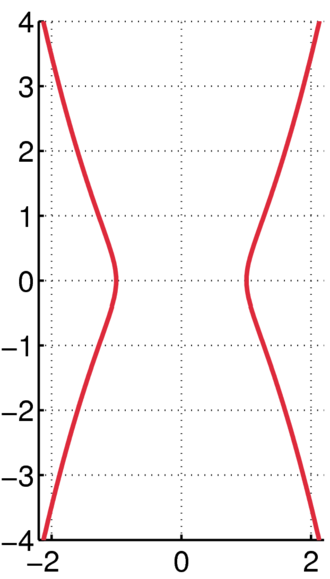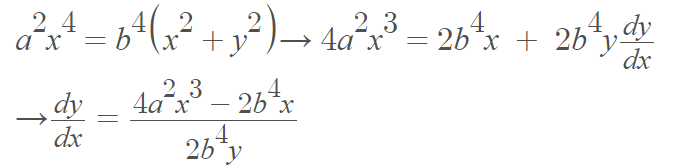# Kampyle of EudoxusGraph of Kampyle of Eudoxus, with a = 1.Kampyle of Eudoxus is a family of curves studied by the Greek mathematician Eudoxus in relation to doubling the cube; Eudoxus, a student of Plato, was famous for finding formulas for measuring cones, and cylinders, and pyramids . He also constructed the first mathematical model of the universe .

The word kamplye (pronounced with three syllables) comes from the Greek word for “crooked staff” .

The general formula for kampyle of Eudoxus is:

a2x4 = b4(x2 + y2)

Where:

• a and b are nonzero constants,
• (x, y) ≠ (0, 0). In other words, the origin is excluded as a solution to the equation.

This is an open curve that never closes or completes. It is an increasing curve—single, non-repeating curvature .

The curve has the polar equation r cos2 θ = a.
The parametric equations are x = a sec(t); y = a tan(t) sec(t), where t ∈ [-π/2, π/2]. The curve has discontinuities at t = ± π/2 .

## Derivative of Kampyle of Eudoxus

The derivative of the curve can be found with implicit differentiation:## Geometry of Kampyle of Eudoxus

• The two intercepts are: (0, a, 0), (π, -a, 0).
• The minimum is at (0, a, 0).
• The maximum is at (π, -a, 0).
• There are four points of inflection: (tan-1 ± (√2)/2, ± a(√6)/2, a(√3)/2 .

## References

 Fifty Famous Curves, Lots of Calculus Questions, And a Few Answers.
 Tan, S. (2020). Handbook of Famous Plane Curves Using Mathematica.
 Schwartzman, S. (1994). The Words of Mathematics. An Etymological Dictionary of Mathematical Terms Used in English. Mathematical Association of America.
 Krawczyk, R. Seashell Interpretation in Architectural Forms.
 Lawrence, J. (2013). A Catalog of Special Plane Curves. Dover Publications.

CITE THIS AS:
Stephanie Glen. "Kampyle of Eudoxus" From StatisticsHowTo.com: Elementary Statistics for the rest of us! https://www.statisticshowto.com/kampyle-of-eudoxus/